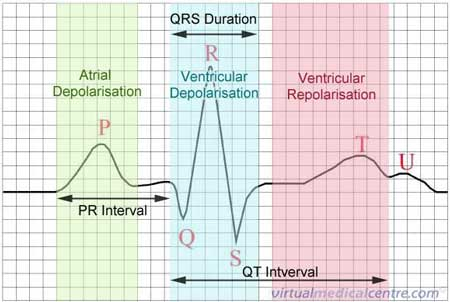# Algorithm/Formula for Measuring Waveform Peaks Needed

Hello,

I'm working on a project and I need help with an algorithm/formula for measuring waveform peaks within a minute. Any peak that is > 4 V. If you need anymore information to help, please ask.

So, 1023 counts in your adc mean 5v so 4v means how many adc counts? DO the maths.
Then just do a thigh loop always reading the adc and record the peak value, easy.

``````Then just do a thigh loop
``````

I looked on the reference page. No thigh loops. What is a thigh loop?

Probably meant tight loop.

And if not, there is the thigh master!

Ok what he is trying to do. we have a ECG Monitor sending an Analog signal to the arduino. the problem we are having is writing an algorithmic that can analyse the QRS and count the R in the signal.Sorry if I misspelled something, mastering two languages is not that easy.
A tight loop is a loop where you only put the necessary code and if possible optimized by hand as much as possible, because if you want to reach the maximum sample rate of the Arduino you will not do things like serial prints that are blocking actions inside the measure loop.

Can you tell more about the duration of the PR interval and the QT interval and the 3 phases?

You might need something like code below ; I added a counter as a wave might have multiple equal peaks in theory. Don;t know if this is tight enough

``````#define SAMPLETIME 60000L    // the L is for Long
int max = 0;
int count = 0;

void setup()
{
Serial.begin(115200);
}

void loop()
{
max = 0;
count = 0;

// WAIT FOR SIGNAL TO START SAMPLING
while (digitalRead(RESETPIN) == LOW) ;   // wait for start - can be done differently tooo

// START SAMPLING
unsigned long startTime = millis()
while (millis() - startTime < SAMPLETIME )
{
if (max == raw)
{
count++;
}
else if (max < raw)
{
max = raw;
count=1;
}
}

// DISPLAY RESULTS
Serial.print("MAX: ");
Serial.println(max);
Serial.print("CNT: ");
Serial.println(count);
}
``````

I looked on the reference page. No thigh loops. What is a thigh loop?

Isn't that a basic movement that most female strippers utilize in their routines?

Lefty

You're gonna need to do some signal conditioning first.
I would sugest running the signal thru a comparator, compare it to a DC level a couple of squares above the P level. Then you would get a nice digital pulse starting on the leading edge of R and you measure the time between the R pulses.
Human heartbeat is pretty low frequency, 60 beats/min resting, 200 bpm for some whose heart is really racing.

Depending on where you set the level, you might be able to get triple pulse too, from P & T also.

robtillaart:
Can you tell more about the duration of the PR interval and the QT interval and the 3 phases?

You might need something like code below ; I added a counter as a wave might have multiple equal peaks in theory. Don;t know if this is tight enough

``````#define SAMPLETIME 60000L    // the L is for Long
``````

int max = 0;
int count = 0;

void setup()
{
Serial.begin(115200);
}

void loop()
{
max = 0;
count = 0;

// WAIT FOR SIGNAL TO START SAMPLING
while (digitalRead(RESETPIN) == LOW) ;   // wait for start - can be done differently tooo

// START SAMPLING
unsigned long startTime = millis()
while (millis() - startTime < SAMPLETIME )
{
if (max == raw)
{
count++;
}
else if (max < raw)
{
max = raw;
count=1;
}
}

// DISPLAY RESULTS
Serial.print("MAX: ");
Serial.println(max);
Serial.print("CNT: ");
Serial.println(count);
}

after testing the program these errors appear.

sketch_jun27a.cpp: In function 'void loop()':
sketch_jun27a:15: error: 'RESETPIN' was not declared in this scope
sketch_jun27a:19: error: expected ',' or ';' before 'while'
sketch_jun27a:38: error: expected `}' at end of input

That's just format issues.
You need to declare the pin in use for RESETPIN before setup, then declare it as input in setup.

starttime is not defined, will probably just treated as 0 unless you give it a value

this needs a ;
unsigned long startTime = millis()

So, some minor tweaks, fix it up & try again.